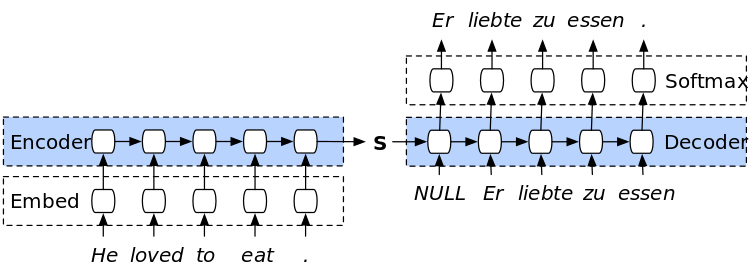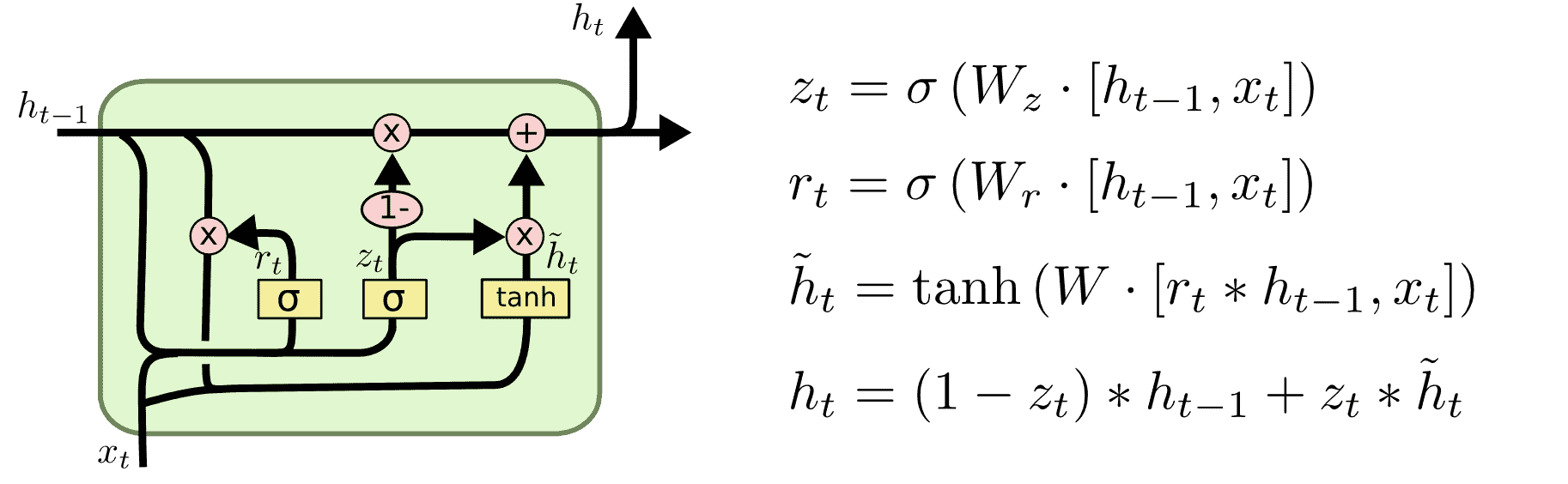# English to German Translation using Seq2Seq Models In PyTorch

Sequence to Sequence models, also referred to as encoder-decoder models, are a family of models that typically train 2 recurrent neural networks. The first RNN, the encoder, is trained to recieve input text and encode it sequentially. The second RNN, the decoder, receives that encoded sequence and performs a mapping to the text. This unique method of training 2 RNNs together was introduced by Cho et al. in https://arxiv.org/pdf/1406.1078v3.pdfand instantly gained popularity in NLP tasks where the input and output are explicit text pairs, such as translation and summarization.

In the following tutorial, we will explore how to create and train Seq2Seq models in PyTorch for English-German translation.

Overview:

• Tokenization
• Creating Encoder RNN
• Creating Decoder RNN
• Setup and Training
• Evaluation

In :
import torch
import torch.nn as nn
import torch.nn.functional as F

import numpy as np

import matplotlib.pyplot as plt


We use the Multi30k dataset, a popular dataset for translations from and to many languages. For our purposes, we use the English to German translation dataset:

https://github.com/multi30k/dataset

In :
train_path_en = "train.lc.norm.tok.en.txt"
train_path_de = "train.lc.norm.tok.de.txt"
test_path_en = "test_2017_flickr.lc.norm.tok.en.txt"
test_path_de = "test_2017_flickr.lc.norm.tok.de.txt"


Before working with PyTorch, make sure to set the device. This line of code selects a GPU if available.

In :
device = torch.device('cuda' if torch.cuda.is_available() else 'cpu')
device

Out:
device(type='cuda')

The data is in txt files, so we use the standard Python open method.

In :
with open(train_path_en) as en_raw_train:
with open(train_path_de) as de_raw_train:
with open(test_path_en) as en_raw_test:
with open(test_path_de) as de_raw_test:


The number of instances in our training and test sets match those mentioned in the Github repository of the dataset.

In :
print(len(en_parsed_train))
print(len(de_parsed_train))
print(len(en_parsed_test))
print(len(de_parsed_test))

29000
29000
1000
1000


We show 5 English-German instances below. The data is preprocessed and semi-tokenized (splitting by spaces is sufficient).

In :
for i in range(5):
print("English: {} \n German: {} \n".format(en_parsed_train[i].strip(), de_parsed_train[i].strip()))

English: two young , white males are outside near many bushes .
German: zwei junge weiße männer sind im freien in der nähe vieler büsche .

English: several men in hard hats are operating a giant pulley system .
German: mehrere männer mit schutzhelmen bedienen ein antriebsradsystem .

English: a little girl climbing into a wooden playhouse .
German: ein kleines mädchen klettert in ein spielhaus aus holz .

English: a man in a blue shirt is standing on a ladder cleaning a window .
German: ein mann in einem blauen hemd steht auf einer leiter und putzt ein fenster .

English: two men are at the stove preparing food .
German: zwei männer stehen am herd und bereiten essen zu .



## Tokenization

Creating tokenized version for all sets by splitting each sentence:

In :
en_train = [sent.strip().split(" ") for sent in en_parsed_train]
en_test = [sent.strip().split(" ") for sent in en_parsed_test]
de_train = [sent.strip().split(" ") for sent in de_parsed_train]
de_test = [sent.strip().split(" ") for sent in de_parsed_test]


Since we have 2 languages in this tutorial, we will create 2 separate dictionaries:

In :
en_index2word = ["<PAD>", "<SOS>", "<EOS>"]

for ds in [en_train, en_test]:
for sent in ds:
for token in sent:
if token not in en_index2word:
en_index2word.append(token)

for ds in [de_train, de_test]:
for sent in ds:
for token in sent:
if token not in de_index2word:
de_index2word.append(token)


Using index2word dictionaries to create the reverse mappings (word2index):

In :
en_word2index = {token: idx for idx, token in enumerate(en_index2word)}
de_word2index = {token: idx for idx, token in enumerate(de_index2word)}


Making sure the mappings are done correctly for both vocabs:

In :
en_index2word

Out:
'a'
In :
en_word2index["a"]

Out:
20
In :
de_index2word

Out:
'ein'
In :
de_word2index["ein"]

Out:
20

Unlike when working with tweets, we cannot simply assume a specific max sequence length. To have a good estimate, we calculate the average lengths of both languages in the training sets.

In :
en_lengths = sum([len(sent) for sent in en_train])/len(en_train)
de_lengths = sum([len(sent) for sent in de_train])/len(de_train)

In :
en_lengths

Out:
13.018448275862069
In :
de_lengths

Out:
12.438137931034483

The average length for english instances is ~13 words and for German instances is ~12 words. We can assume that most instances are less than 20 words long, and use that as an upper bound for padding and truncating.

In :
seq_length = 20

In :
def encode_and_pad(vocab, sent, max_length):
sos = [vocab["<SOS>"]]
eos = [vocab["<EOS>"]]

if len(sent) < max_length - 2: # -2 for SOS and EOS
n_pads = max_length - 2 - len(sent)
encoded = [vocab[w] for w in sent]
else: # sent is longer than max_length; truncating
encoded = [vocab[w] for w in sent]
truncated = encoded[:max_length - 2]
return sos + truncated + eos


Created fixed-size tokenized sets:

In :
en_train_encoded = [encode_and_pad(en_word2index, sent, seq_length) for sent in en_train]
en_test_encoded = [encode_and_pad(en_word2index, sent, seq_length) for sent in en_test]
de_train_encoded = [encode_and_pad(de_word2index, sent, seq_length) for sent in de_train]
de_test_encoded = [encode_and_pad(de_word2index, sent, seq_length) for sent in de_test]


Finally for data preparation, we create the PyTorch Datasets and DataLoaders required:

In :
batch_size = 50

train_x = np.array(en_train_encoded)
train_y = np.array(de_train_encoded)
test_x = np.array(en_test_encoded)
test_y = np.array(de_test_encoded)

train_ds = TensorDataset(torch.from_numpy(train_x), torch.from_numpy(train_y))
test_ds = TensorDataset(torch.from_numpy(test_x), torch.from_numpy(test_y))

train_dl = DataLoader(train_ds, shuffle=True, batch_size=batch_size, drop_last=True)
test_dl = DataLoader(test_ds, shuffle=True, batch_size=batch_size, drop_last=True)


## Encoder GRUThe Gated Recurrent Unit (GRU) is an RNN that is more efficient than LSTM in handling memory and has very similar performance. We use the GRU as a base model for both the encoder and the decoder.

In :
class EncoderRNN(nn.Module):
def __init__(self, input_size, hidden_size):
super(EncoderRNN, self).__init__()
self.hidden_size = hidden_size

# Embedding layer

# GRU layer. The input and output are both of the same size
#  since embedding size = hidden size in this example
self.gru = nn.GRU(hidden_size, hidden_size, batch_first=True)

def forward(self, input, hidden):
# The inputs are first transformed into embeddings
embedded = self.embedding(input)
output = embedded

# As in any RNN, the new input and the previous hidden states are fed
#  into the model at each time step
output, hidden = self.gru(output, hidden)
return output, hidden

def initHidden(self):
# This method is used to create the innitial hidden states for the encoder


## Decoder GRU

In :
class DecoderRNN(nn.Module):
def __init__(self, hidden_size, output_size):
super(DecoderRNN, self).__init__()
self.hidden_size = hidden_size

# Embedding layer

# The GRU layer
self.gru = nn.GRU(hidden_size, hidden_size)

# Fully-connected layer for scores
self.out = nn.Linear(hidden_size, output_size)

# Applying Softmax to the scores
self.softmax = nn.LogSoftmax(dim=1)

def forward(self, input, hidden):
# Feeding input through embedding layer
output = self.embedding(input)

# Applying an activation function (ReLu)
output = F.relu(output)

# Feeding input and previous hidden state
output, hidden = self.gru(output, hidden)

# Outputting scores from the final time-step
output = self.softmax(self.out(output))

return output, hidden

# We do not need an .initHidden() method for the decoder since the
#  encoder output will act as input in the first decoder time-step


## Setup and Training

In :
hidden_size = 128


Initialing encoder and decoder and sending to device.

In :
encoder = EncoderRNN(len(en_index2word), hidden_size).to(device)
decoder = DecoderRNN(hidden_size, len(de_index2word)).to(device)

In :
encoder

Out:
EncoderRNN(
(gru): GRU(128, 128, batch_first=True)
)
In :
decoder

Out:
DecoderRNN(
(gru): GRU(128, 128)
(out): Linear(in_features=128, out_features=19138, bias=True)
(softmax): LogSoftmax(dim=1)
)

When training Seq2Seq models, you need 2 optimizers, one for the encoder and one for the decoder. They are trained simultaneously with each batch.

In :
criterion = nn.CrossEntropyLoss()
enc_optimizer = torch.optim.Adam(encoder.parameters(), lr = 3e-3)
dec_optimizer = torch.optim.Adam(decoder.parameters(), lr = 3e-3)

In :
losses = []

In :
input_length = target_length = seq_length

SOS = en_word2index["<SOS>"]
EOS = en_word2index["<EOS>"]

epochs = 15

for epoch in range(epochs):
for idx, batch in enumerate(train_dl):

# Creating initial hidden states for the encoder
encoder_hidden = encoder.initHidden()

# Sending to device
encoder_hidden = encoder_hidden.to(device)

# Assigning the input and sending to device
input_tensor = batch.to(device)

# Assigning the output and sending to device
target_tensor = batch.to(device)

# Feeding batch into encoder
encoder_output, encoder_hidden = encoder(input_tensor, encoder_hidden)

# This is a placeholder tensor for decoder outputs. We send it to device as well
dec_result = torch.zeros(target_length, batch_size, len(de_index2word)).to(device)

# Creating a batch of SOS tokens which will all be fed to the decoder
decoder_input = target_tensor[:, 0].unsqueeze(dim=0).to(device)

# Creating initial hidden states of the decoder by copying encoder hidden states
decoder_hidden = encoder_hidden

# For each time-step in decoding:
for i in range(1, target_length):

# Feed input and previous hidden states
decoder_output, decoder_hidden = decoder(decoder_input, decoder_hidden)

# Finding the best scoring word
best = decoder_output.argmax(1)

# Assigning next input as current best word
decoder_input = best.unsqueeze(dim=0)

# Creating an entry in the placeholder output tensor
dec_result[i] = decoder_output

# Creating scores and targets for loss calculation
scores = dec_result.transpose(1, 0)[1:].reshape(-1, dec_result.shape)
targets = target_tensor[1:].reshape(-1)

# Calculating loss
loss = criterion(scores, targets)

# Performing backprop and clipping excess gradients
loss.backward()

enc_optimizer.step()
dec_optimizer.step()

# Keeping track of loss
losses.append(loss.item())
if idx % 100 == 0:
print(idx, sum(losses)/len(losses))

0 9.90767765045166
100 5.055438830120729
200 4.651930824441103
300 4.473189581272214
400 4.34943013714436
500 4.269323982878359
0 4.217673528214945
100 4.145541447374789
200 4.086785013330731
300 4.040421336787784
400 3.9981875188732245
500 3.9589146581873864
0 3.9339153998684617
100 3.894374151063476
200 3.861103242499963
300 3.831441003439118
400 3.8052173178597646
500 3.781167105261925
0 3.765156625400392
100 3.736955089066613
200 3.71116592735436
300 3.6905379418004443
400 3.6711687419192915
500 3.652108652684264
0 3.6390171910811477
100 3.6166391808944316
200 3.5967761984140045
300 3.57911565421147
400 3.5621807050371994
500 3.5473335627671125
0 3.536280471593994
100 3.5173204429782814
200 3.500763186713412
300 3.4849407036801274
400 3.4706644610324364
500 3.457996690949774
0 3.4484520466702313
100 3.431305566336049
200 3.416630296854829
300 3.4031371001496074
400 3.3914639844135106
500 3.380093869956945
0 3.3713394718433185
100 3.3561761766579026
200 3.3432564499847657
300 3.331156344021222
400 3.319945334105501
500 3.3098122236682146
0 3.30249308373645
100 3.289580716233896
200 3.2782820600341407
300 3.267122483596076
400 3.2569476834035918
500 3.2477239301014076
0 3.2403265840818634
100 3.228343153600293
200 3.2178457707326102
300 3.208032793636837
400 3.199034264197534
500 3.190811839642964
0 3.1844970692932306
100 3.1739695379237487
200 3.1641688918177433
300 3.1551276574543587
400 3.1471946279073295
500 3.139633842998602
0 3.133628665681656
100 3.124281754778447
200 3.115111059338973
300 3.107178645582903
400 3.099718079702672
500 3.092298934390735
0 3.086908446185771
100 3.077929504463683
200 3.0698084278223883
300 3.0626272860349597
400 3.0556491855499037
500 3.0492099774553285
0 3.0442397899233113
100 3.036150526148598
200 3.0285960513019945
300 3.021938648672071
400 3.015707957310755
500 3.00929181400245
0 3.0048252632429766
100 2.997475309436331
200 2.990660230509723
300 2.984362547576831
400 2.978542374761546
500 2.9727385375549784


## Evaluation

We can see that the loss is decreasing steadily as training proceeds, which means the model is learning the task correctly.

In :
plt.plot(losses)

Out:
[<matplotlib.lines.Line2D at 0x7f7b54873790>]Testing with an example sentence:

In :
test_sentence = "the men are walking in the streets ."

# Tokenizing, Encoding, transforming to Tensor

In :
encoder_hidden = torch.zeros(1, 1, hidden_size)
encoder_hidden = encoder_hidden.to(device)

input_tensor = test_sentence.to(device)

result = []

encoder_outputs = torch.zeros(seq_length, encoder.hidden_size, device=device)

encoder_output, encoder_hidden = encoder(input_tensor, encoder_hidden)

dec_result = torch.zeros(target_length, 1, len(de_index2word)).to(device)

decoder_input = torch.tensor([SOS]).unsqueeze(dim=0).to(device)
decoder_hidden = encoder_hidden
for di in range(1, target_length):
decoder_output, decoder_hidden = decoder(decoder_input, decoder_hidden)
best = decoder_output.argmax(1)
result.append(de_index2word[best.to('cpu').item()])
if best.item() == EOS:
break

decoder_input = best.unsqueeze(dim=0)
dec_result[di] = decoder_output

scores = dec_result.reshape(-1, dec_result.shape)
targets = target_tensor.reshape(-1)

In :
" ".join(result)

Out:
'die männer laufen durch die straßen . . <EOS>'

You can use Google Translate to verify the translation if you do not know German. Also, experiment with different example sentences to check model behavior in different situations.